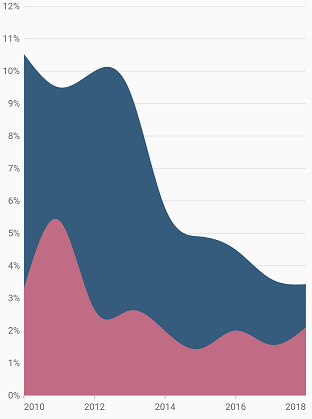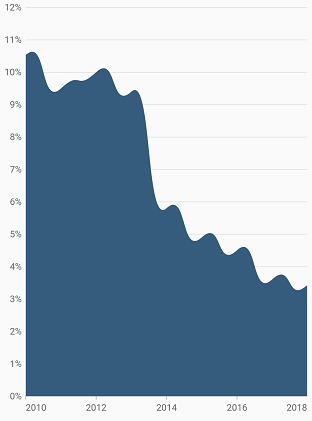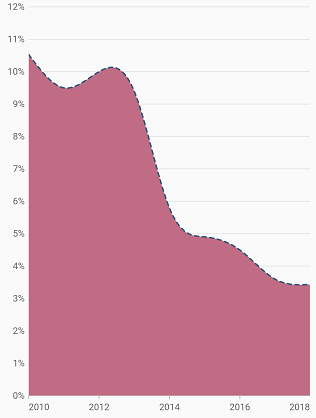# Spline area Chart in Flutter Cartesian Charts (SfCartesianChart)

11 Apr 202212 minutes to read

To render a spline area chart, create an instance of `SplineAreaSeries`, and add it to the `series` collection property of `SfCartesianChart`. The following properties can be used to customize the appearance of spline area chart:

``````@override
Widget build(BuildContext context) {
final List<ChartData> chartData = <ChartData>[
ChartData(2010, 10.53, 3.3),
ChartData(2011, 9.5, 5.4),
ChartData(2012, 10, 2.65),
ChartData(2013, 9.4, 2.62),
ChartData(2014, 5.8, 1.99),
ChartData(2015, 4.9, 1.44),
ChartData(2016, 4.5, 2),
ChartData(2017, 3.6, 1.56),
ChartData(2018, 3.43, 2.1),
];
return Scaffold(
body: Center(
child: SfCartesianChart(
series: <ChartSeries>[
SplineAreaSeries<ChartData, int>(
dataSource: chartData,
xValueMapper: (ChartData data, _) => data.x,
yValueMapper: (ChartData data, _) => data.y
),
SplineAreaSeries<ChartData, int>(
dataSource: chartData,
xValueMapper: (ChartData data, _) => data.x,
yValueMapper: (ChartData data, _) => data.y1
),
]
)
)
);
}
class ChartData {
ChartData(this.x, this.y, this.y1);
final int x;
final double y;
final double y1;
}``````## Spline area rendering types

The `splineType` allows you to change the spline area curve in series. The following types can be used in `SplineAreaSeries`:

• natural
• monotonic
• cardinal
• clamped

By default, the value of `splineType` is `natural`.

The following code sample demonstrates how to set the `splineType` value to `cardinal`. When you set the cardinal type, you can specify the desired line tension of the `cardinal` spline using the `cardinalSplineTension` property. The value of this property ranges from 0 to 1.

``````@override
Widget build(BuildContext context) {
final List<ChartData> chartData = <ChartData>[
ChartData(2010, 10.53),
ChartData(2011, 9.5),
ChartData(2012, 10),
ChartData(2013, 9.4),
ChartData(2014, 5.8),
ChartData(2015, 4.9),
ChartData(2016, 4.5),
ChartData(2017, 3.6),
ChartData(2018, 3.43),
];
return Scaffold(
body: Center(
child: Container(
child: SfCartesianChart(
series: <ChartSeries>[
SplineAreaSeries<ChartData, int>(
dataSource: chartData,
splineType: SplineType.cardinal,
cardinalSplineTension: 0.9,
xValueMapper: (ChartData data, _) => data.x,
yValueMapper: (ChartData data, _) => data.y
)
]
)
)
)
);
}``````## Dashed spline area

The `dashArray` property of the `SplineAreaSeries` is used to render spline area series with dashes. Odd value is considered as rendering size and even value is considered as gap.

``````@override
Widget build(BuildContext context) {
final List<ChartData> chartData = <ChartData>[
ChartData(2010, 10.53),
ChartData(2011, 9.5),
ChartData(2012, 10),
ChartData(2013, 9.4),
ChartData(2014, 5.8),
ChartData(2015, 4.9),
ChartData(2016, 4.5),
ChartData(2017, 3.6),
ChartData(2018, 3.43),
];
return Scaffold(
body: Center(
child: Container(
child: SfCartesianChart(
series: <ChartSeries>[
SplineAreaSeries<ChartData, int>(
dataSource: chartData,
dashArray: <double>[5, 5],
xValueMapper: (ChartData data, _) => data.x,
yValueMapper: (ChartData data, _) => data.y
)
]
)
)
)
);
}``````